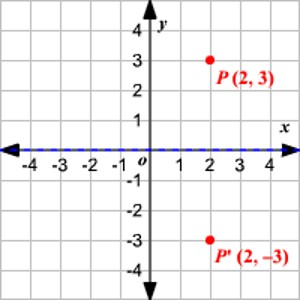Reflection of a Point in the x-axis

A reflection can be thought of as folding or “flipping” an object over the line of reflection.

When you reflect a point across the x-axis, the x-coordinate remains the same, but the y-coordinate is transformed into its opposite (its sign is changed). If you forget the rules for reflections when graphing, simply fold your paper along the x-axis (the line of reflection) to see where the new figure will be located.

Reflection in the line y = 0 i.e., in the x-axis.

The line y = 0 means the x-axis.

Let P be a point whose coordinates are (x, y).

Let the image of P be P’ in the axis.

Clearly, P’ will be similarly situated on that side of OX which is opposite to P. So, the y-coordinates of P’ will be – y while its x-coordinates will remain same as that of P.

The image of the point (x, y) in the x-axis is the point (x, -y).

Symbolically, Mxx (x, y) = (x, -y)

Rules to find the reflection of a point in x-axis:

1. Retain the abscissa i.e. x-coordinate.
2. Change the sign of ordinate i.e., y-coordinate.

Therefore, when a point is reflected in the x-axis, the sign of its ordinate changes.

Examples:

(i) The image of the point (3, 4) in the x-axis is the point (3, -4).

(ii) The image of the point (-3, -4) in the x-axis is the point (-3, -(-4)) i.e., (-3, 4).

(iii) The reflection of the point (5, -7) in the x-axis = (5, 7) i.e., Mxx (5, -7) = (5, 7)

(iv) The reflection of the point (9, 0) in the x-axis is the point itself, therefore, the point (9, 0) is invariant with respect to x-axis.

(v) The reflection of the point (-a, -b) in the x-axis = (-a, b) i.e., Mxx (-a, -b) = (-a, b)Solved examples to find the reflection of a point in the x-axis

prob-1: Find the points onto which the points (11, -8), (-6, -2) and (0, 4) are mapped when reflected in the x-axis.

Solution:

We know that a point (x, y) maps onto (x, -y) when reflected in the x-axis. So, (11, -8) maps onto (11, 8); (-6, -2) maps onto (-6, 2) and (0, 4) maps onto (0, -4).

prob-2: Which of the following points (-2, 0), (0, -5), (3, -3) are invariant points when reflected in the x-axis?

Solution:

We know that only those points which lie on the line are invariant points when reflected in the line. So, only those points are invariant which lie on the x-axis. Hence, the invariant points must have y-coordinate = 0. Therefore, only (-2, 0) is the invariant point.

Information Source: